# Find the work W done by the 18-newton force

Find the work W done by the 18-newton force.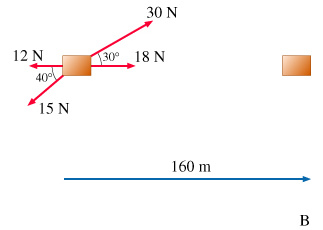Concepts and reason
The concept of work done and force is required to solve the problem.
The work done by the applied force is calculated by taking the dot product of force and displacement.

Fundamentals
Work is done whenever force acting on an object causes it to displace in the direction of force.
The work done by a constant force is determined by the following expression: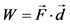Here, F is the magnitude of the force acting on the object and d is the displacement of the object.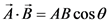Dot product between two vector quantities is equal to,

The expression for work done is,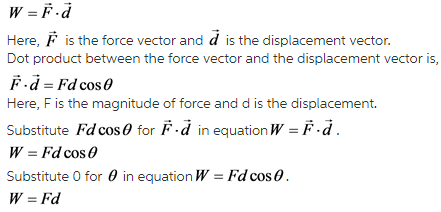Explanation:
The force of 18 N acting on the object is in the direction of displacement. Hence, the angle between the force and displacement is zero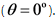The expression for work done is,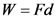Here, F is the magnitude of force and d is the displacement.
Substitute 18 N for F and 160 m for d in equationdetermine the work done.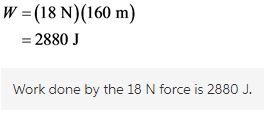Explanation:
Work done by a constant force is equal to the product of the magnitude of the force and the displacement of the object in the direction of force.